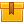# Measurement

## Prerequisites

A basic understanding of electronic devices and circuits and familiarity with the various oscilloscope functions, probes, and controls, as well as knowledge of waveform
types is required.

## Description

This is the final lesson in the iKNOW Oscilloscopes Library. This lesson teaches how to determine various measurements taken with an oscilloscope.

## Objectives

Measure voltage using a waveform. Measure the period and frequency of a waveform. Define pulse rise time and pulse width. Measure rise time. Measure pulse width. Measure phase shift.

## ContentThis is the final lesson in the iKNOW™ Oscilloscopes Library. This
lesson teaches how to determine various measurements taken with an
oscilloscope.

•Oscilloscopes Measurement

0
0 Reviews